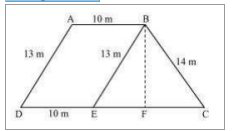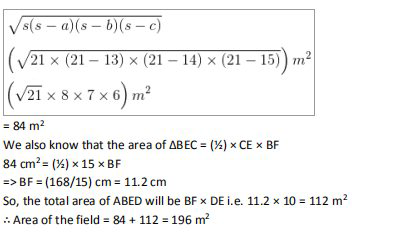# NCERT Solutions Class 9 Mathematics Solutions for Heron’s Formula - Exercise 12.2 in Chapter 12 - Heron’s Formula

Question 9 Heron’s Formula - Exercise 12.2

A field is in the shape of a trapezium whose parallel sides are 25 m and 10 m. The non

parallel sides are 14 m and 13 m. Find the area of the field.

First, draw a line segment BE parallel to the line AD. Then, from B, draw a perpendicular on the

line segment CD.Now, it can be seen that the quadrilateral ABED is a parallelogram. So,

AB = ED = 10 m

AD = BE = 13 m

EC = 25 - ED = 25 - 10 = 15 m

Now, consider the triangle BEC,

Its semi perimeter (s) = (13+ 14 + 15)/2 = 21 m

By using Heron’s formula,

Area of ΔBEC =Related Questions

Lido

Courses

Teachers

Book a Demo with us

Syllabus

Maths
CBSE
Maths
ICSE
Science
CBSE

Science
ICSE
English
CBSE
English
ICSE
Coding

Terms & Policies

Selina Question Bank

Maths
Physics
Biology

Allied Question Bank

Chemistry
Connect with us on social media!